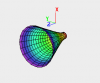# Simple code for Parametric Surface Wave of Revolution

Generating a parametric wave for display of a Gizmo surface generally involves several explicit code loops (See the Mobius pxp in the Visualization examples). A parametric wave is usually required for complicated surfaces, multi-valued surfaces, or those not easily expressed as z(x,y). One somewhat simpler case is a surface of revolution that has axial symmetry around a 'z' axis. This allows generation of the parametric wave using only simple wave operations, without for-endfor loops. An example is given to show the method:
Function makeSurface()  //  make a parametric surface of revolution for Gizmo display

make/O/N=(20,21)           wC, wS, wZ
setscale/P x, 0, 0.025,"", wC, wS, wZ  //  'x' here is the spatial 'z'(axial) direction
setscale/I  y, 0, 2*pi,"",    wC, wS, wZ  //  'y' here is the angular variable
wC = RofZ(x)*cos(y)  // will be parametric x coordinate
wS = RofZ(x)*sin(y)   // will be parametric y coordinate
wZ =  x                    // parametric z coordinate; now have 3 2-D waves
Make/O/N=(20,21,3) wSurf
Concatenate /O  {wC, wS, wZ}, wSurf  //  promoted 3rd parametric dimension is x,y,z space
End

Function RofZ(x)  // 1-D function example for surface radius vs axial coordinate
variable x

variable w0 = 0.006, Zr = 0.075
return w0*sqrt(1+(x/Zr)^2)
End

The code can be generalized by using FUNCREF identification from several radial profile functions. I am attaching a Gizmo snapshot from the above code. Users may wish to do their own lateral and axial Gizmo display scaling. I have not previously seen the use of Concatenate in this context. I apologize (to AG?) if I am mistaken.ForumSupportGallery